Goal Seek Excel

A what-if analysis tool used to find the value of an assumption based on the desired output

What is the Goal Seek Excel Tool?

The Goal Seek Excel tool is a form of what-if analysis that tells us what value an assumption needs to be in order to reach a desired output or result. It is a form of reverse engineering, where the user starts with the outcome and answer that they want and Excel works backward to find out what’s required. It is commonly used in financial modeling and other types of financial analysis.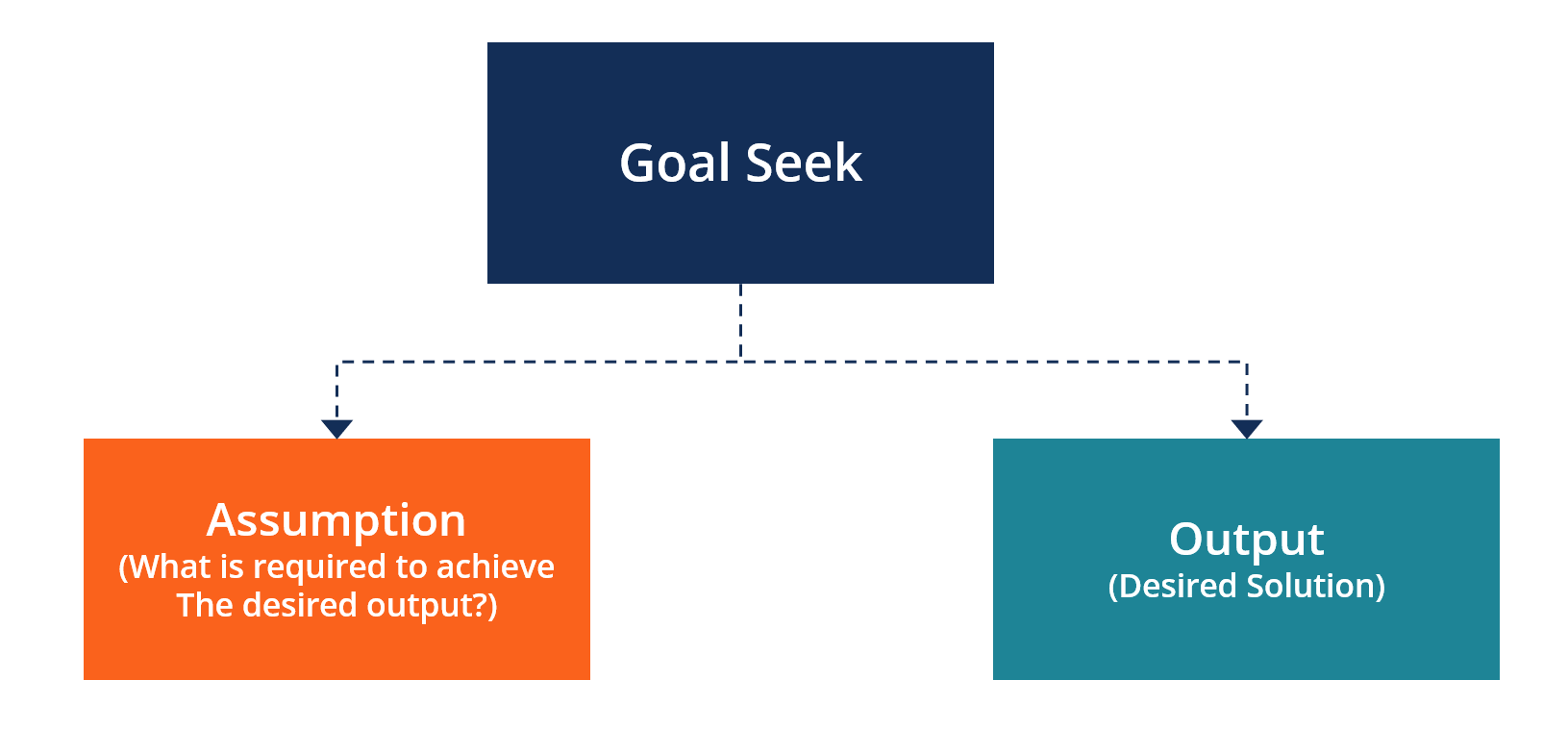How to Use the Goal Seek Excel Tool (Example)

Follow this step-by-step guide on how to use Goal Seek to understand the cause and effect relationships between inputs and outputs in an Excel model:

Step #1

Navigate to the cell that contains the output or result you want to analyze. In this case, we have a simple model that examines how much profit/loss a shoe retailer makes depending on how many shoes they sell. We want to see how many shoes they need to sell to break even (generate zero profit). To run this test, we put the cursor in cell C8.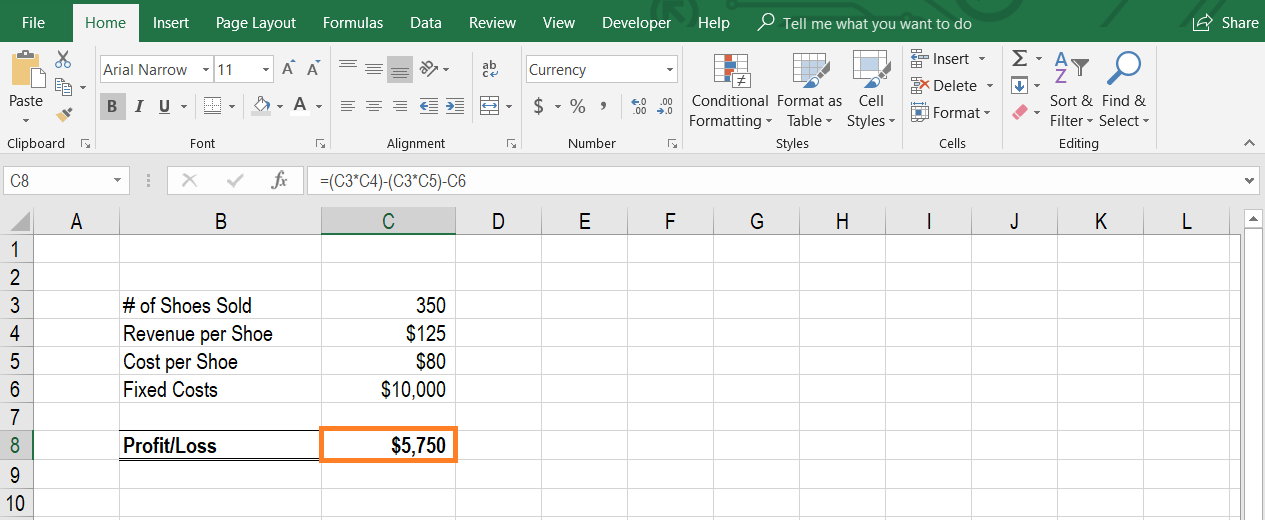Step #2

Open Goal Seek by accessing the Data section of the ribbon, then select What-If Analysis and then click Goal Seek. The keyboard shortcut on Windows is Alt, A, W, G. When this is completed the Goal Seek Excel dialogue box will appear.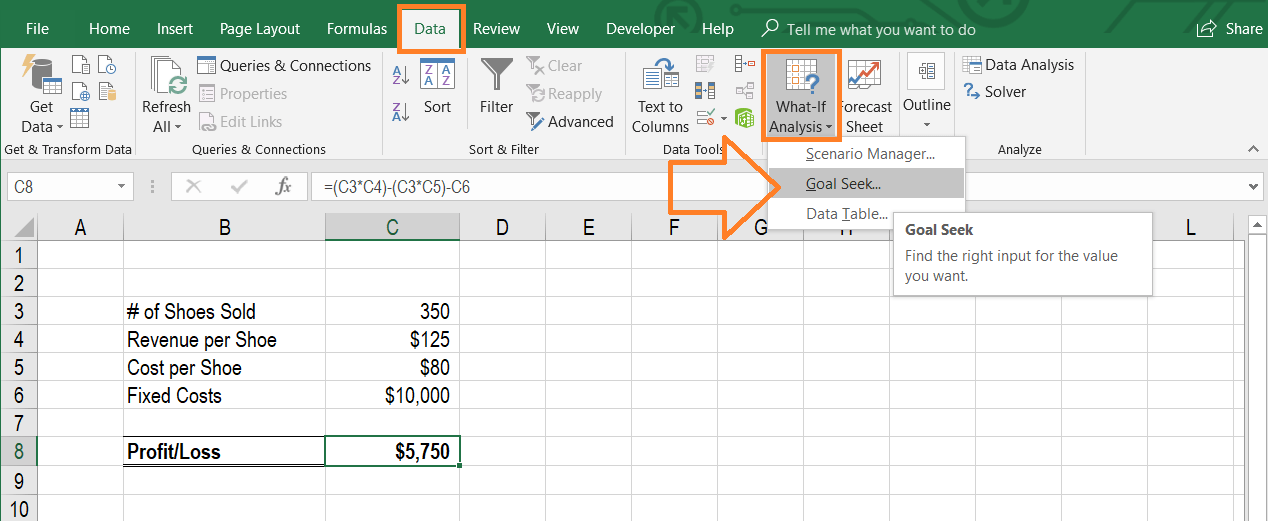Step #3

When the Goal Seek Excel dialogue box appears, link the cell that you want to set the value of and then enter the value you want it to be set to. In this case, we want to change the profit/loss value to zero. It means linking the Set Cell to C8 and the To Value to 0.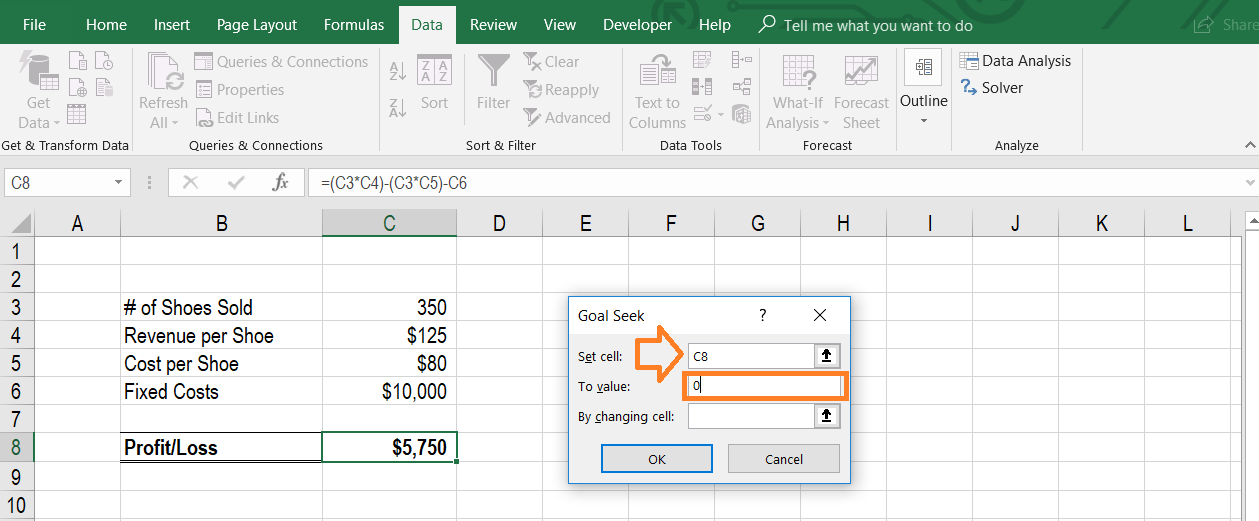Step #4

Link to the cell that you want Excel to change in order to generate the solution. Since we want to know how many shoes need to be sold in order to break even, we link the By Changing Cell to be C3. Then press OK.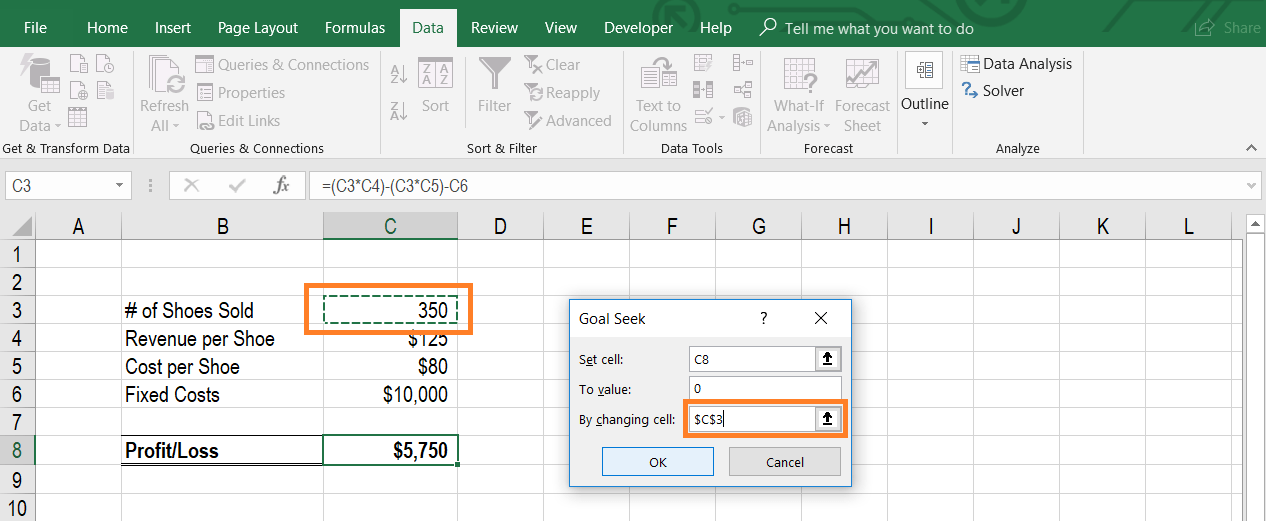Step #5

At this point, the solution will be displayed in the By Changing Cell and the Set Value Cell. In this case, we see that 222 shoes need to be sold (cell C3) to break-even with zero profit (cell C8).

If you wish to leave the results of the test in the spreadsheet, press OK. Note, this will change your model to now have the new values in it. If you simply wanted to take note of the solution, but return to your original numbers, press Cancel (this is the most common way to use Goal Seek).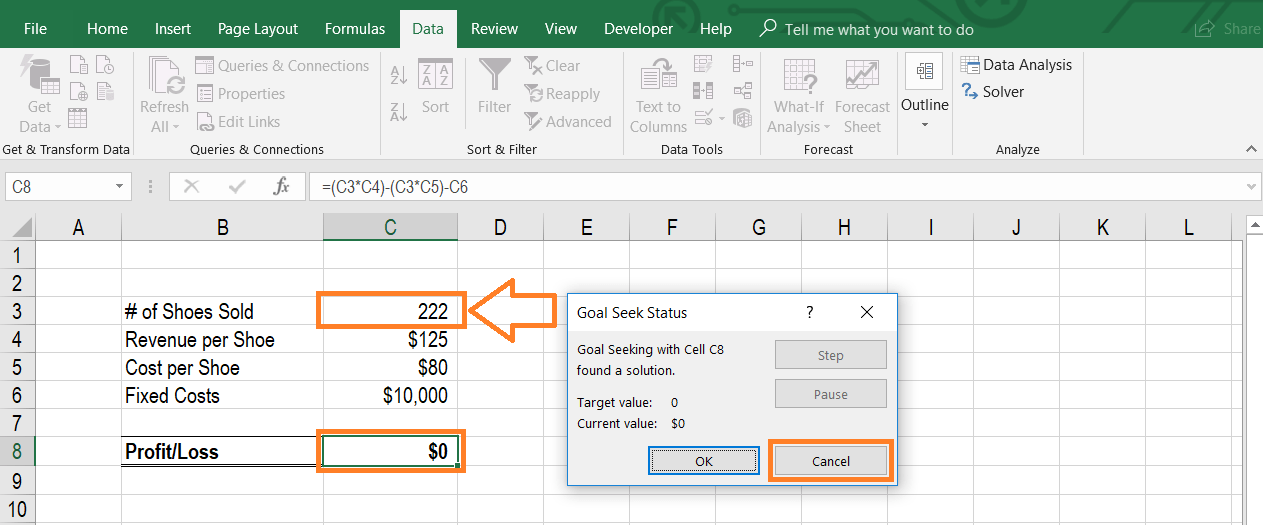Why Use the Goal Seek Excel Function?

There are many reasons to use the Goal Seek Excel function, especially when performing financial modeling and analysis.

Common reasons include:

• Break-even analysis
• Scenario analysis
• Sensitivity analysis
• Understanding cause and effect
• Answering specific questions (e.g., how many shoes do we need to sell to earn \$2,500 in profit?)
• Stress testing a model

Video Explanation of Goal Seek

Below is a short video that will show you exactly how to use this function in your spreadsheets. Watch the demonstration and start using the function on your own.

Thank you for reading CFI’s guide to the Goal Seek Excel function, how to use it, and why it matters. To continue learning and advancing your career as a financial analyst, you will find these additional resources helpful.